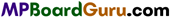These MP Board Class 11th Chemistry Notes for Chapter 10 The s-Block Elements help students to get a brief overview of all the concepts.

## MP Board Class 11th Chemistry Notes Chapter 2 Structure of Atom

→ General electronic configuration of j-block elements is ns1-2.

→ IA group elements are called Alkaline earth metals. Its general electronic configuration is ns1.

→ Order of basicity of oxides : Li2O < Na2O, K2O < Rb2O < Cs2O.→ Order of stability of carbonate : Li2CO3 < Na2CO3< K2CO3 < Rb2CO3 < Cs2CO3.

→ s-block elements are metals and strongly electropositive.

→ 2nd group elements are known as Alkaline earth metals. Their general electronic configuration is ns2.
Oxidation number of Alkali metals is +1 and oxidation number of Alkaline earth metals is +2.

→ s-block elements are strongly reducing.

→ Li represents diagonal relationship with Mg and Be with Al.

→ Carbonates of Alkali metals are soluble in water whereas Alkaline earth metals are insoluble in water.

→ Alkali metals combine with halogens to form halides, these are ionic crystalline solids. Sodium is a highly reactive metal, it is stored in kerosene oil.

→ Alkali metals are paramagnetic but their compounds are diamagnetic.

→ Magnesium sulphate is known as Epsom salt.

→ Calcium sulphate is known as Gypsum salt.→ Order of stability of metal hydroxides is as follows :
CsOH > RbOH > KOH > NaOH > LiOH
Ba(OH)2 > Sr(OH)2 > Ca(OH)2 > Mg(OH)2

→ Order of stability of IInd group metal carbonates is as follows :
BeCO3 < MgCO3 < CaCO3 < SrCO3

→ Order of stability of halides is as follows : .
MF > MCI > MBr > MI

→ Order of stability of metal sulphates is as follows :
BeSO4 > MgSO4 > CaSO4 > SrSO4 > BaSO4

→ Density of Alkali metals decreases from Li to Cs. Though weight of K < weight of Na. → Hydration energy of Alkali metal decreases on moving down in the group. → Its value increases from left to right in a period. → Heat of hydration of IInd group > Heat of hydration of Ist group.

→ Order of conductivity of ions of Alkali metals in aqueous solution is as follows :
Li+ < Na+ < K+ < Rb+ < Cs+ < Fr+

→ Although ionization potential of Li is highest, still due to very high hydration energy, it is a strong reducing agent.→ Compounds of Alkaline earth metals are less ionic than the compounds of Alkali metals.

→ Solution of Alkali metals in liquid ammonia, is a conductor of electricity. Blue colour of the solution is due to ammoniated electrons. In concentrated solution, blue colour changes to bronze colour.

→ Alkali metals form normal oxides, peroxides and superoxides. Their order of stability is Normal oxide > Peroxide > Superoxide

→ Oxides of Alkali metals react with water to form alkali metal hydroxides. Due to high hydration energy these are soluble in water and large amount of energy is released.

→ Na2CO3 is known as soda ash and Na2CO3.10H2O is known as washing soda or salt soda. It is manufactured by Solvay process.

→ 8% NaCl (according to mass) aqueous solution is known as brine.

→ NaHCO3 is known as Baking soda.

→ Some difference in properties of Alkaline earth metals with Alkali metals is due to their small atomic or ionic radius and high positive charge.

→ Plaster of Paris (POP) [CaSO4. $$\frac { 1 }{ 2 }$$ H2O] is obtained on heating Gypsum(CaSO4.2H2O) to 120°C.→ Normal composition of Portland cement is 70% CaO, 18% SiO2, 5% Al2O3, 3% Fe2O3 and 2% MgO. To slow down its setting process some amount of gypsum is mixed in it.